## 1. Co-teaching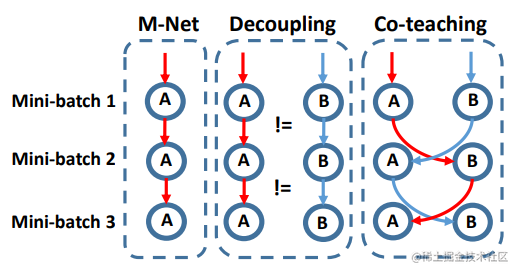1. 为什么根据一个动态的 $R(T)$来采样低loss的样本可以帮助我们找到干净的样本？
2. 为什么需要两个网络并交叉更新参数？

【参考：】 paper

## 2. 置信学习

1. Count，估计噪声标签与真实标签的联合分布
2. Clean，清理错误样本。
3. Re-Training，清理之后，调整样本类别权重，使用Co-teaching重新训练。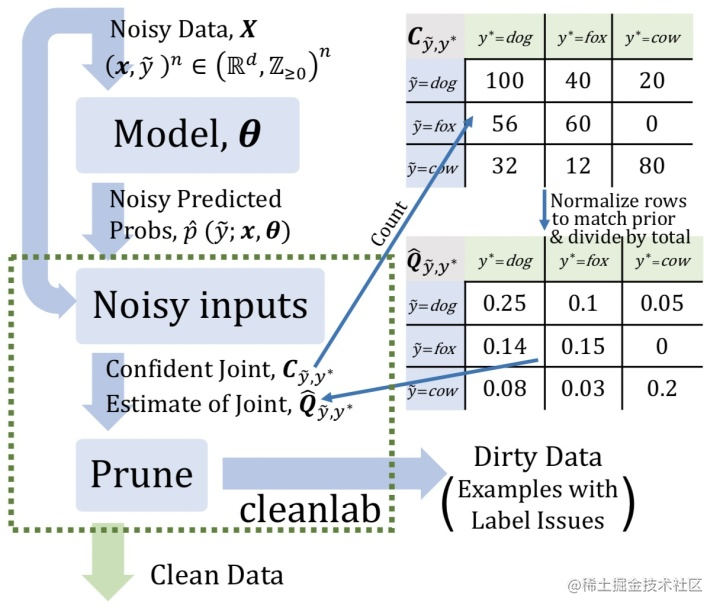1.Count

• step1，交叉验证
1. 首先通过交叉验证，在数据集中计算第 $i$个样本在第 $j$个类别下的概率： $P[i][j]$
2. 计算每个人工标注类别 $j$下的平均概率 $t[j]$，作为置信度阈值
3. 最后确定样本 $i$的真实标签。,其真实标签 $y^*$ $j$个类别中的最大概率 $argmax_jP[i][j]$,并且需要满足 $P[i][j]$ > $t[j]$
• step2，计算计数矩阵 $C_{\hat{y},y^*}$（类似于混淆矩阵）。如图1中， $C_{\hat{y}=dog,y^=fox*}=40$，表示x人工标记为dog但实际为fox的样本为40个。算法如下：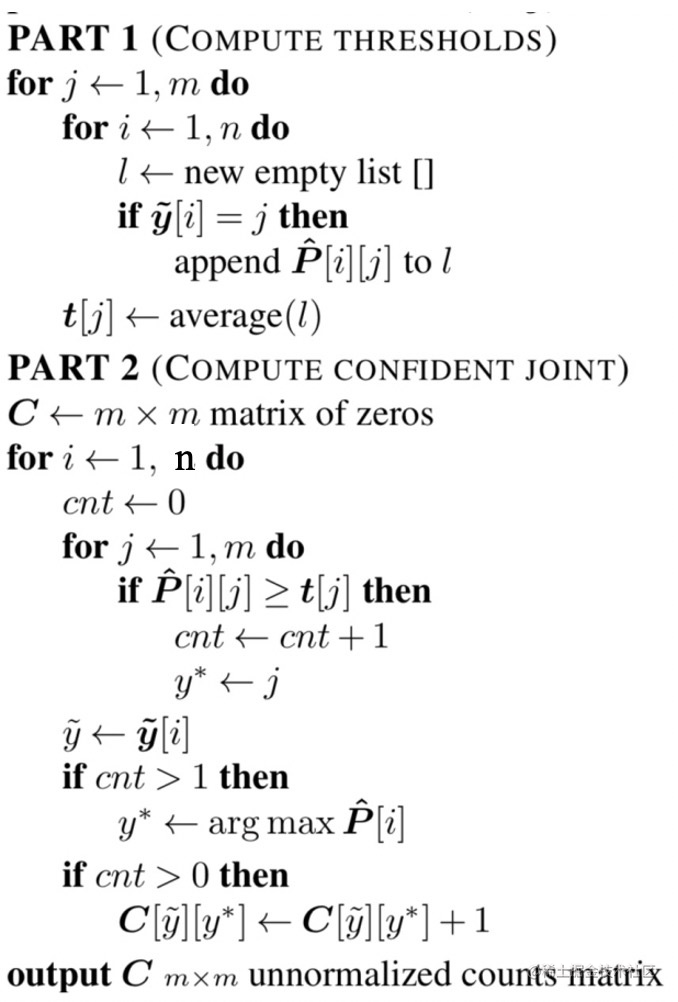• step3, 计算矫正的计数矩阵,因为在上一步的计算中，有一个条件是需要大于t[j]，所以计数矩阵 $C_{\hat{y},y^*}$和是小于样本总数的。所以这一步的目的就是为了让计数总和与人工标记的样本总数相同。其中， $X_{\hat{y}=i}$表示人工标注标签 $\hat{y} = i$的样本总数。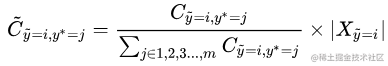• step 4: 计算联合分布 $Q_{\hat{y},y^*}$，就是用上面的结果除以样本总数：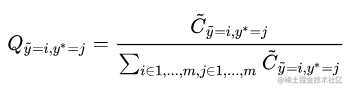2.Clean

• M1 $C_{confusion}$,选取 $\hat{y} != argmax_{i\in{1,2,...m}}{P[i][j]}$的样本过滤，即对于样本 $i$,如果人工标注标签与模型预测最大值标签不相同则过滤。
• M2: 选取构造计数矩阵 $C_{\hat{y},y^*}$过程中，进入费对角单元的样本进行过滤。
• M3: Prune by Class (PBC) ,对于每一个类别 $i\in{1...m}$选择 $n.\sum_{j\in{1..m:j!=i}}{C_{\hat{y}=i,y^*=j}[i]}$个样本,其中n是样本总数，按照 $P[i][j]$排序，过滤到得分最低的。
• M4:Prune by Noise Rate (PBNR) ,对于非对角单元，选取 $n.Q_{\hat{y}=i,y^*=j}$个样本进行过滤，按照 $P[\hat{y}=i]$- $P[\hat{y}=j]$排序，过滤到得分最低的。

3.Re-Training

【参考：】 cleanlab 参考1

(待续...)